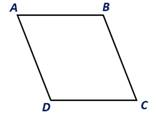Chapter 4.1, Problem 12EElementary Geometry For College St...

7th Edition
Alexander + 2 others
ISBN: 9781337614085

Solutions

Chapter
SectionElementary Geometry For College St...

7th Edition
Alexander + 2 others
ISBN: 9781337614085
Textbook Problem

Given that m ∠ A = 2 x 5 , and m ∠ B = x 2 , find the measure ofeach angle of. ▱ A B C D .To determine

To find:

The measure of each angle of ABCD.

Explanation

Given:

Given that Given that mA=2x5, and mB=x2, and the ABCD as shown below.

Corollary:

1. The opposite angles of a parallelogram are congruent.

2. Two consecutive angles of a parallelogram are supplementary.

Description:

In the given parallelogram ABCD, given that Given that mA=2x5, and mB=x2.

As per the corollary that the two consecutive angles of a parallelogram are supplementary, the sum of the angles mA and mB is 180°.

To find the value of x, substitute mA=2x5, and mB=x2 in mA+mB=180°

2x5+x2=180°

4x+5x10=180°

9x10=180°

x=180

Still sussing out bartleby?

Check out a sample textbook solution.

See a sample solution

The Solution to Your Study Problems

Bartleby provides explanations to thousands of textbook problems written by our experts, many with advanced degrees!

Get Started

let f(x) = x3 + 5, g(x) = x2 2, and h(x)= 2x + 4. Find the rule for each function. 3. fg

Applied Calculus for the Managerial, Life, and Social Sciences: A Brief Approach

Evaluate the integral. 1/21/241x2dx

Calculus: Early Transcendentals

Differentiate the function. p(v)=lnv1v

Single Variable Calculus: Early Transcendentals, Volume I

Differentiate the function. f(t) = 1.4t5 2.5t2+ 6.7

Single Variable Calculus: Early Transcendentals

True or False: is a geometric series.

Study Guide for Stewart's Single Variable Calculus: Early Transcendentals, 8th